# Measurement I Units of Measurement w Number vs

• Slides: 12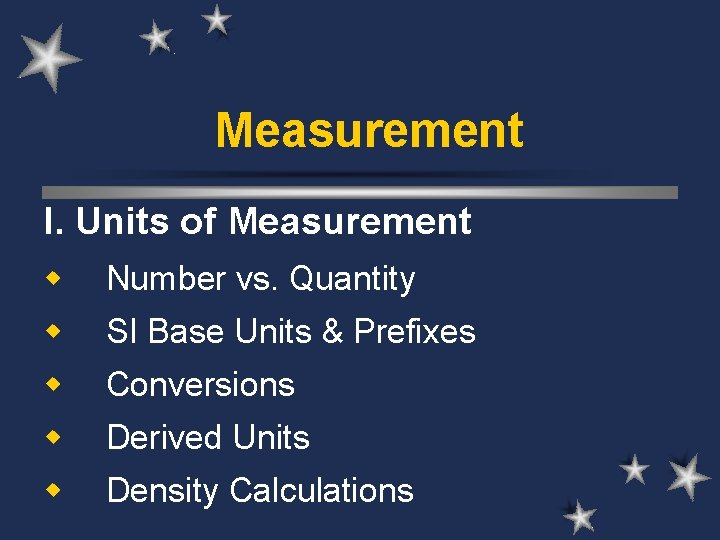Measurement I. Units of Measurement w Number vs. Quantity w SI Base Units & Prefixes w Conversions w Derived Units w Density Calculations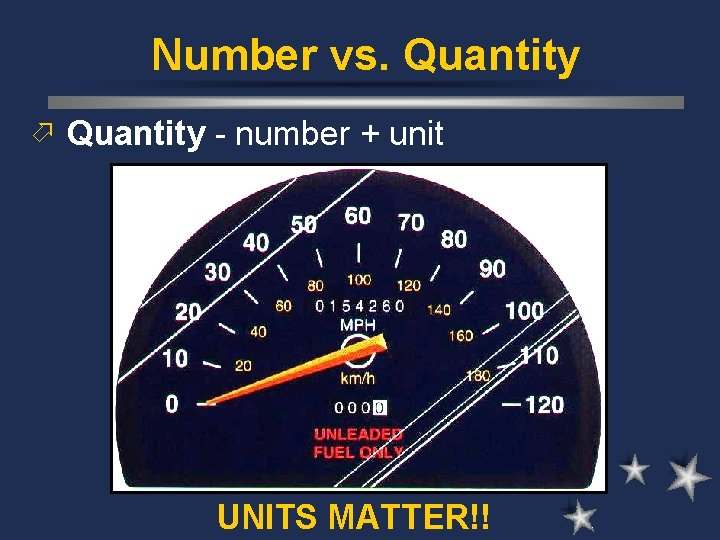Number vs. Quantity ö Quantity - number + unit UNITS MATTER!!SI Units Quantity Base Unit Symbol meter m Mass kilogram kg Time second s Temp kelvin K ampere A Length CurrentSI Units Prefix Symbol Factor megakilodecicentimillimicronanopico- M k d c m n p 106 103 10 -1 10 -2 10 -3 10 -6 10 -9 10 -12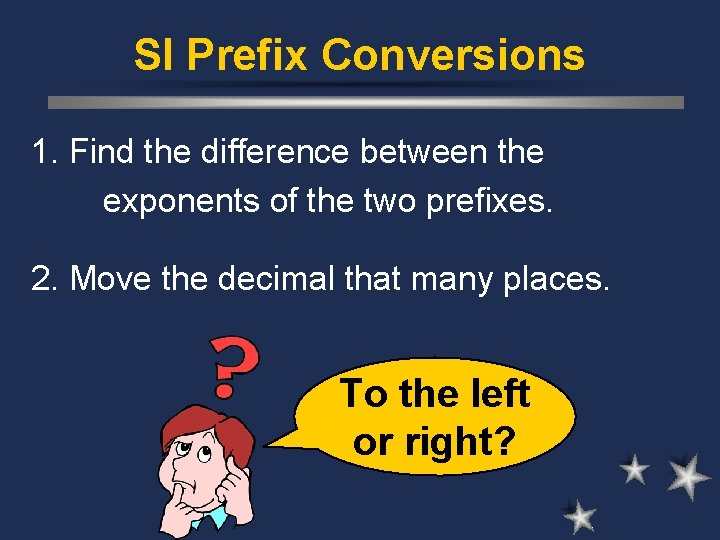SI Prefix Conversions 1. Find the difference between the exponents of the two prefixes. 2. Move the decimal that many places. To the left or right?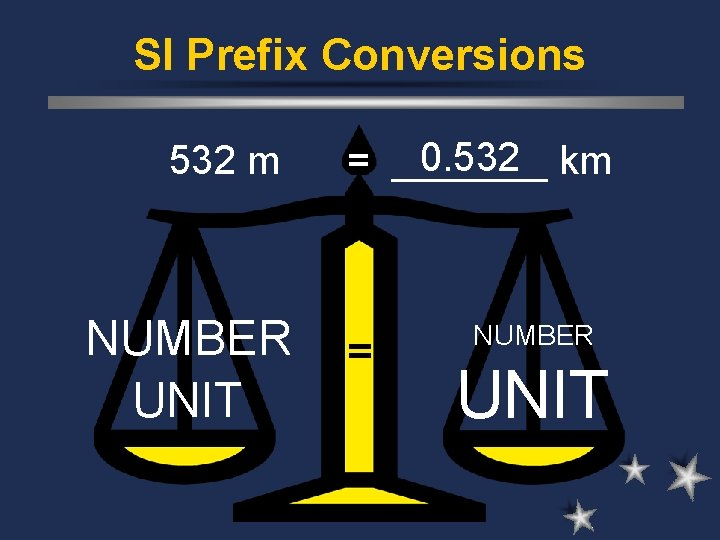SI Prefix Conversions 532 m NUMBER UNIT 0. 532 km = _______ = NUMBER UNITmove right move left SI Prefix Conversions Prefix Symbol Factor megakilodecicentimillimicronanopico- M k d c m n p 106 103 10 -1 10 -2 10 -3 10 -6 10 -9 10 -12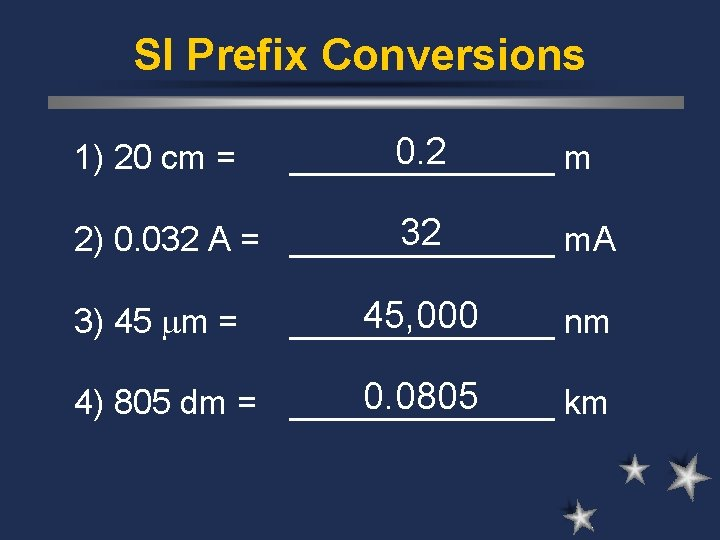SI Prefix Conversions 1) 20 cm = 0. 2 _______ m 32 2) 0. 032 A = _______ m. A 3) 45 m = 45, 000 _______ nm 0. 0805 4) 805 dm = _______ kmDerived Units ö Combination of base units. ö Volume - length 1 cm 3 = 1 m. L 1 dm 3 = 1 L ö Density - mass per unit volume (g/cm 3) M D= V M D V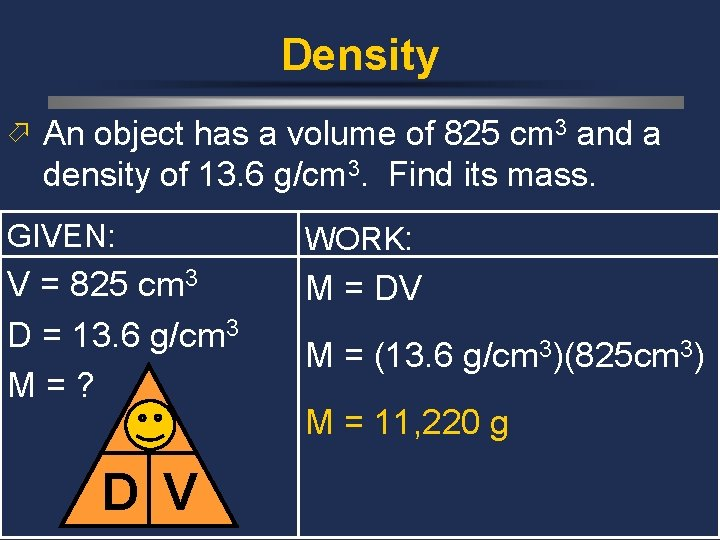Density ö An object has a volume of 825 cm 3 and a density of 13. 6 g/cm 3. Find its mass. GIVEN: WORK: V = 825 cm 3 D = 13. 6 g/cm 3 M=? M = DV M D V M = (13. 6 g/cm 3)(825 cm 3) M = 11, 220 g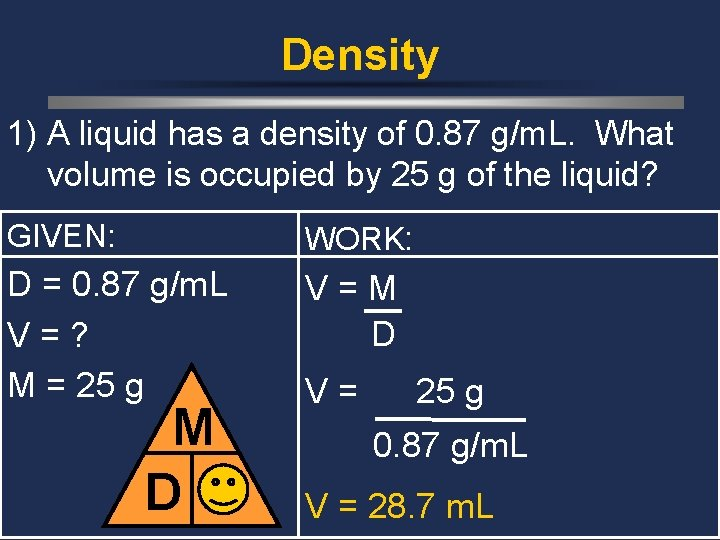Density 1) A liquid has a density of 0. 87 g/m. L. What volume is occupied by 25 g of the liquid? GIVEN: WORK: D = 0. 87 g/m. L V=? M = 25 g V=M D V V= 25 g 0. 87 g/m. L V = 28. 7 m. LDensity 2) You have a sample with a mass of 620 g & a volume of 753 cm 3. Find density. GIVEN: WORK: M = 620 g V = 753 cm 3 D=? D=M V M D V D= 620 g 753 cm 3 D = 0. 82 g/cm 3